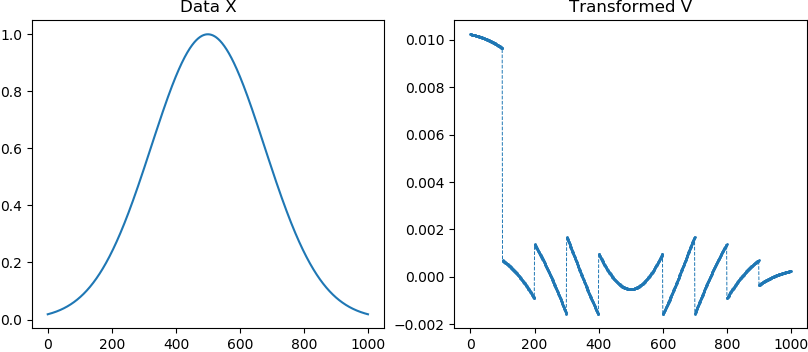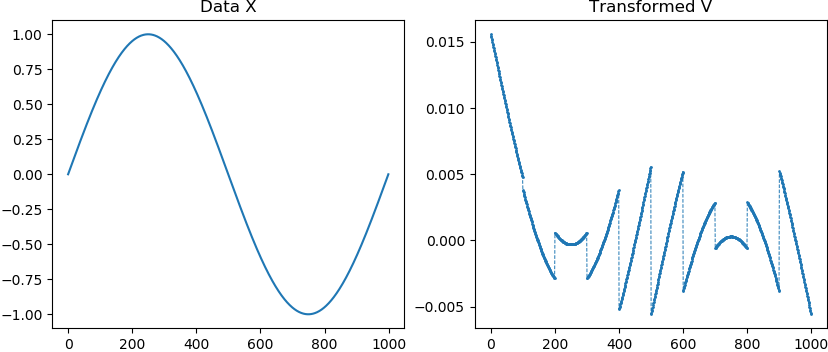# #StackBounty: #data-transformation #interpretation #transform #underdetermined How to interpret a specific data transformation?

### Bounty: 100

I came across this specific data transformation in the context of a physics application, which by itself is rather complex and hence out of the scope of this question. However since this transformation is applicable to general data sets, I wondered if there’s a way to describe general properties of or to interpret this specific data transformation.

Suppose a set of $$N$$ data points $${x_1, ldots, x_N}$$ where $$N = mcdot n$$ for two integer numbers $$m, n > 1$$. Then the transformation from $$x_i$$ to $$v_i$$ is given by the following system of linear equations (with $$m+1$$ rows and $$mcdot n$$ columns):

$$begin{pmatrix} 1 & cdots & 1 & 0 & cdots & 0 & cdotscdots & 0 & cdots & 0 \ 0 & cdots & 0 & 1 & cdots & 1 & cdotscdots & 0 & cdots & 1 \ vdots & & vdots & vdots & & vdots & & vdots & & vdots \ 0 & cdots & 0 & 0 & cdots & 0 & cdotscdots & 1 & cdots & 1 \ x_{1} & cdots & x_{n} & x_{n+1} & cdots & x_{2n} & cdotscdots & x_{(m-1)cdot n+1} & cdots & x_{mcdot n} end{pmatrix} cdot begin{pmatrix} v_1 \ vdots \ vdots \ vdots \ v_{mcdot n}$$

# end{pmatrix}

begin{pmatrix}
1 \
0 \
vdots \
0 \
0
end{pmatrix}
\$\$

The first $$m$$ rows of the above coefficient matrix $$A$$ can be described as follows:

$$A_{i,j} = begin{cases} 1 quad textrm{if} ;; (i-1)cdot n < j leq icdot n \ 0 quad textrm{otherwise} end{cases}$$

for $$i, j in {1, ldots N}$$.
The last row of $$A$$ simply holds all the data points $$x_i$$.
The r.h.s. vector $$b$$ is all zeros except for the first row which equals $$1$$ (in fact any other row except the last one could be chosen to hold the $$1$$; it’s important that there is only one non-zero entry though).

This represents an underdetermined system of linear equations for which a solution can be found by minimizing the L2-norm. The solution $$V^{ast}$$ is then given by the following $$Ntimes N$$ system of equations:

$$A^T A V^{ast} = A^T b$$

where $$A^T$$ is the transpose of $$A$$ and $$b$$ is the r.h.s. vector of the original system of equations. The solution $$V^{ast}$$ can be found as the least-squares solution by pseudo-inversion of the matrix $$A^T A$$ via singular value decomposition.

Now what I’m interested in are general properties of this transformation and how the transformed data $$v_i$$ reflects the original data $$x_i$$. Even when describing all of the involved steps, I’m having difficulties interpreting the results.

## Example application

This is some sample Python code which applies the above transformation to different data sets (Gaussian, sin, cos, exp).

``````import matplotlib.pyplot as plt
import numpy as np

m = 10
n = 100
N = m*n

x = np.exp(-np.linspace(-2, 2, N)**2)
# x = np.sin(np.linspace(0, 2*np.pi, N))
# x = np.cos(np.linspace(0, 2*np.pi, N))
# x = np.exp(-np.linspace(0, 4, N))

A = np.zeros((m+1, N))
for i in range(m):
A[i, i*n:(i+1)*n] = 1
A[-1, :] = x

b = np.zeros(m+1)
b = 1

v, *info = np.linalg.lstsq(A, b, rcond=None)

fig, (ax1, ax2) = plt.subplots(ncols=2, figsize=(10, 4))
ax1.set_title('Data X')
ax1.plot(x)
ax2.set_title('Transformed V')
ax2.plot(v, '--o', lw=0.7, ms=1)

plt.show()
``````

### Output for Normal Distribution### Output for Sine Function### Output for Cosine Function### Output for Exponential Function

Get this bounty!!!

This site uses Akismet to reduce spam. Learn how your comment data is processed.